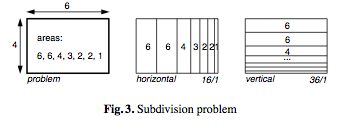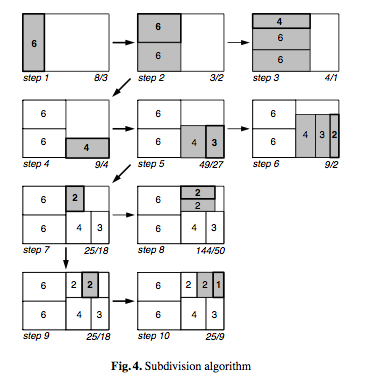画面を分割していく手法 まとめ

Treemap

こういうやつ。Treemaps for space-constrained visualization of hierarchies

Treemapの歴史。有名所がまとまってる。
まずはここを見ると良いかもしれない。

Squarified Treemaps

Treemapの各領域を正方形に近づけたやつThe first step of our algorithm is to split the initial rectangle. We choose for a hori- zontal subdivision, because the original rectangle is wider than high. We next fill the left half. First we add a single rectangle (figure 4). The aspect ratio of this first rectangle is 8/3. Next we add a second rectangle, above the first. The aspect ratios improve to 3/2. However, if we add the next (area 4) above these original rectangles, the aspect ratio of this rectangle is 4/1. Therefore, we decide that we have reached an optimum for the left half in step two, and start processing the right half.
The initial subdivision we choose here is vertical, because the rectangle is higher than wide. In step 4 we add the rectangle with area 4, followed by the rectangle with area 3 in step 5. The aspect ratio decreases. Addition of the next (area 2) however does not improve the result, so we accept the result of step 5, and start to fill the right top partition.newsmapNewsmap is an application that visually reflects the constantly changing landscape of the Google News news aggregator.

まだ読んでる途中

あとでもっと追加する予定。